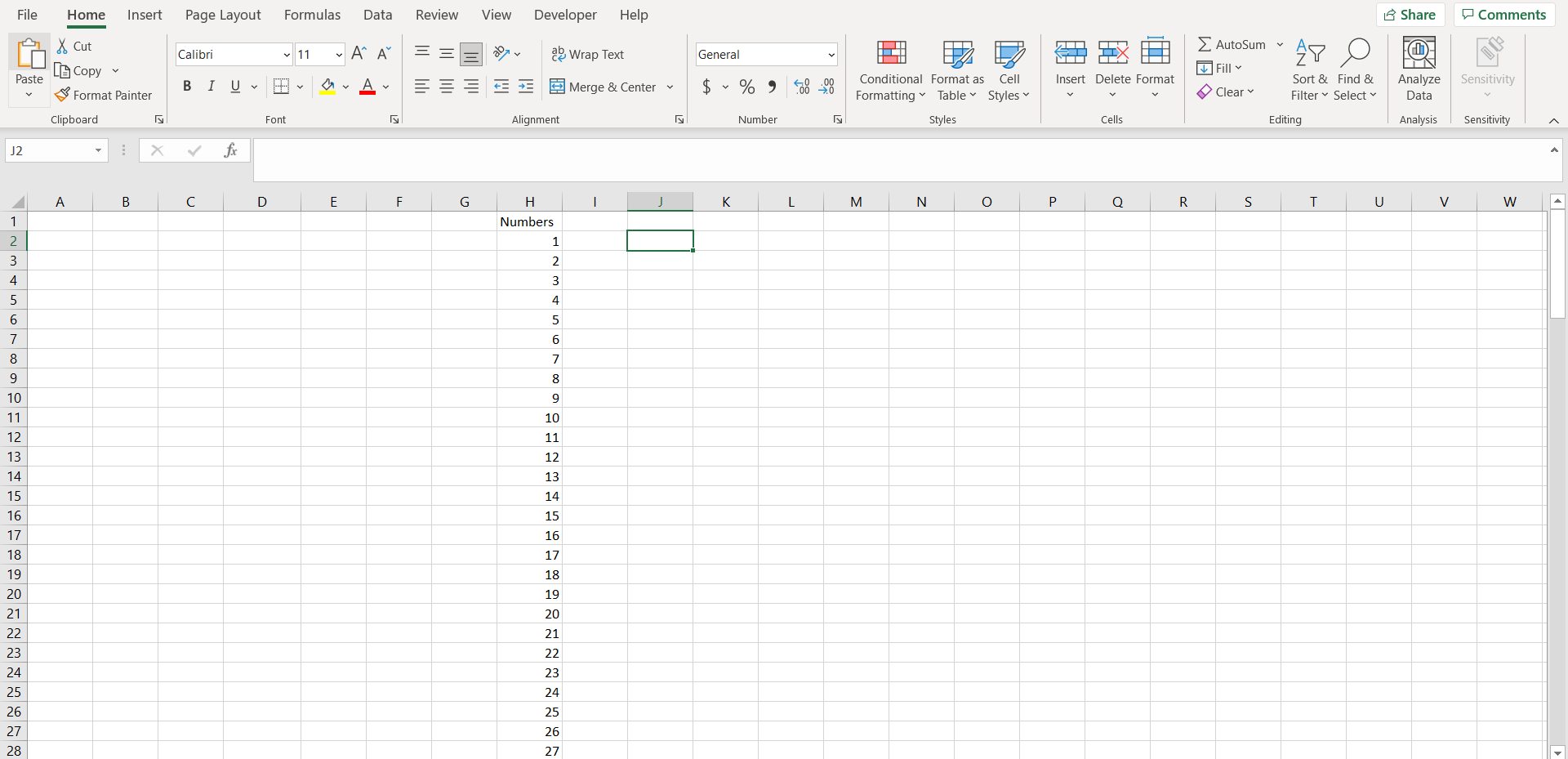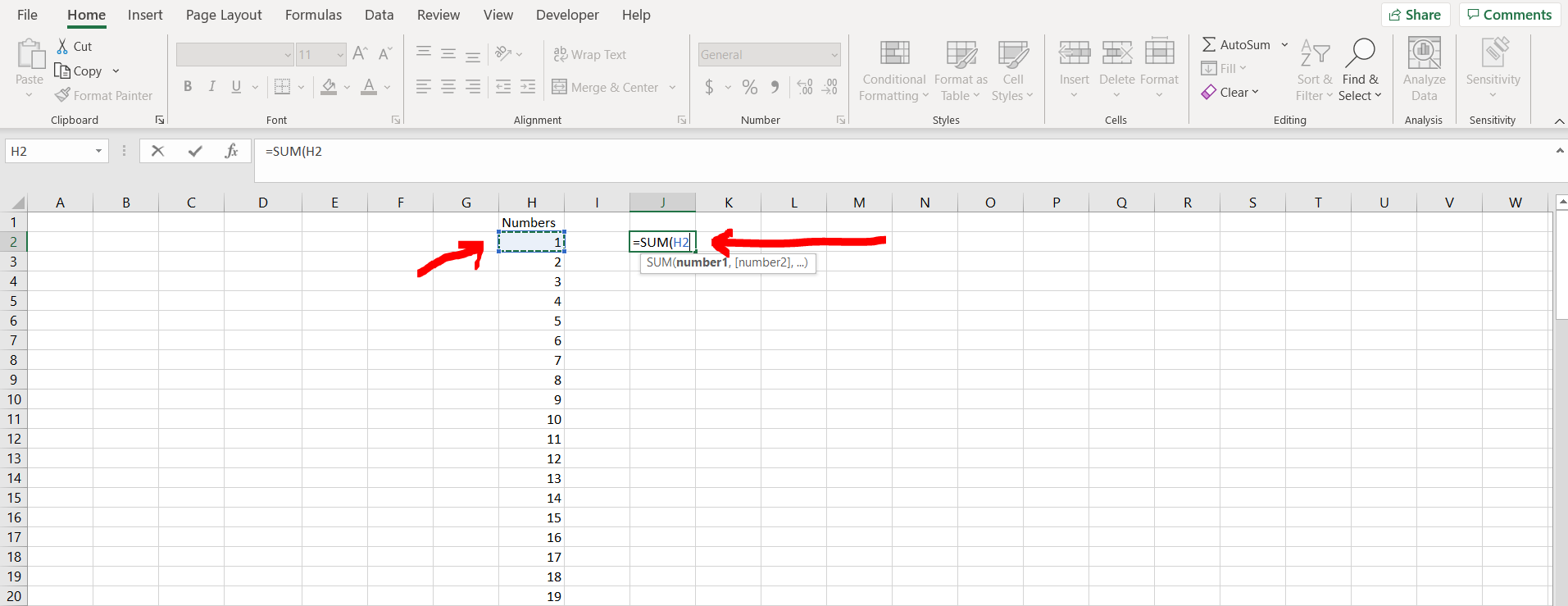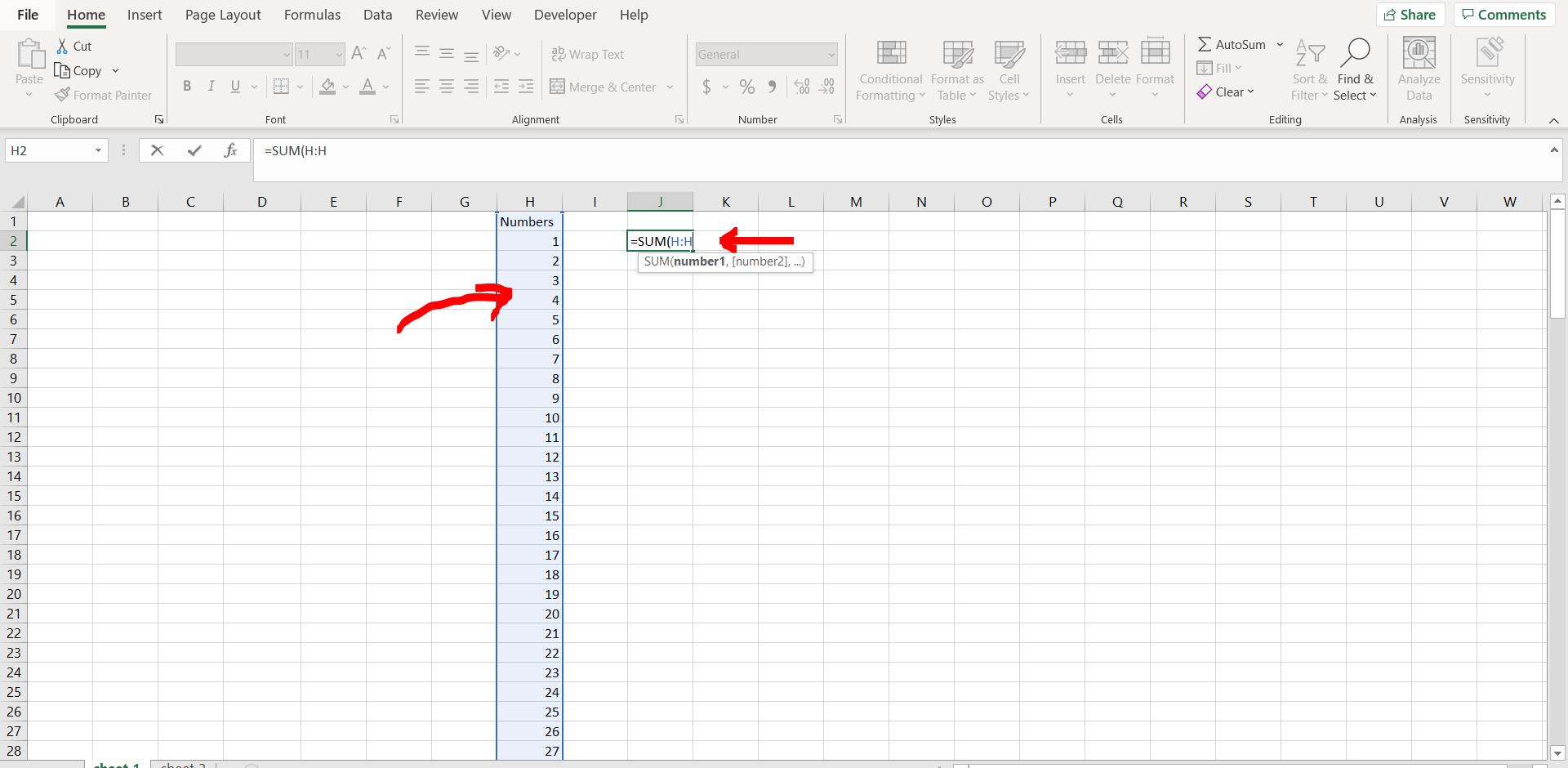# How to add all the numbers in a column in ExcelExcel offers a very interesting way to add all the numbers in a column. We can cater to this problem statement by using the “SUM()” formula. We can perform the below mentioned way to add all the numbers in a column in excel:

### Step 1- Excel workbook with a column with numbers– Open the desired Excel workbook which contains a column populated with some numbers

### Step 2 – Entering the formula– Now in any cell type “=”, and then type “SUM(“. Now click on any cell in the column where the numbers are present. (We have selected cell “H2”)

### Step 3 – Select the entire column– Now click “CTRL + SPACEBAR”. This will select the entire column as shown in the image above. Now press “Enter”.

### Step 4 – Sum obtained– We can see the sum of the entire column now.